Technical Information Site of Power Supply Design

2021.06.09 DC/DC

# Resistance and Inductance of Copper Foil

PCB Layout of a Step-Up DC/DC Converter

This article explains the resistance and inductance of copper foil. The comments here are not limited to the case of step-up DC/DC converters, but apply to PCBs in general, and so should be considered part of basic knowledge of PCB layout.

Resistance of Copper Foil

The copper foil that is metal trace on a PCB has electrical resistance. When a large current flows, conduction losses result in a voltage drop and heat generation. A wiring line in which large currents flow must be studied to determine the resistance value of the copper foil.

Normally, the resistance of copper foil is considered in area units. The following graph shows the resistance value per unit area of copper foil. Here the resistance values are for general copper foil of thickness 35 μm, width 1 mm, and length 1 mm. The resistance value can be calculated using the formula below.Using the resistance value per unit area RP read off from the graph, the formula can be modified as follows. For example, we see that at 25°C, the resistance value of copper foil of width 3 mm and length 50 mm is 8.17 mΩ, as indicated by the equation on the lower right.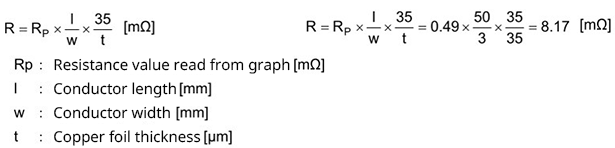In this example, the voltage drop when a 3 A current is flowing is 24.5 mV. From the graph, when the temperature rises to 100°C, RP increases to 0.63 mΩ, and the voltage drop increases to 31.6 mV. This is an increase of about 29%. When such a voltage drop due to copper foil cannot be allowed, in essence, the wiring width is examined.

Inductance of Copper Foil

Copper foil has an inductance. The inductance of copper foil can be represented by the following formula. A graph is also shown that plots the inductance obtained using this formula when, for wiring widths of 0.2 mm to 10 mm, the wiring length is changed.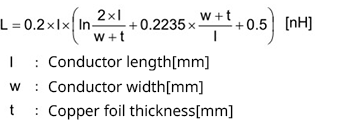From the equation, we see that the inductance hardly depends at all on the copper foil thickness. Moreover, from the graph it is clear that even when the wiring width is doubled, there is surprisingly little decrease in the inductance. Consequently, we see that in order to suppress the inductance of copper foil, shortening the wiring length is effective.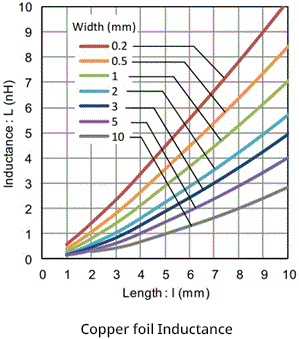If the current flowing in printed wiring with inductance L (H) changes by i (A) over time t (s), then the following voltage appears across the ends of the printed wiring.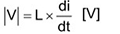For example, when a 2 A current flows for 10 ns in printed wiring with an inductance of 6 nH, the voltage generated is as follows.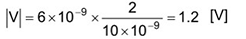The parasitic inductance of wiring not only generates large voltages under some conditions to affect operation, but in some cases can cause component failure, and so appropriate caution is necessary.

#### Key Points:

・The resistance of copper foil appears as a drop in voltage, and depends on the temperature.

・The inductance of copper foil can result in high voltages under some circumstances, thus requiring caution.

・Shortening wiring lengths is effective to reduce inductances.